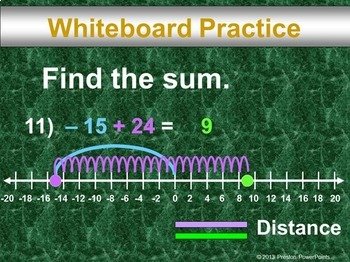# (7th) Adding Integers with a Number Line in a PowerPoint PresentationSubject
Resource Type
File Type
Zip (26 MB|*62)
Standards
\$3.00
• Product Description
• Standards

7th Grade Math Adding Integers with a Number Line in a PowerPoint Presentation

This slideshow lesson is very animated with a flow-through technique. I developed the lesson for my 7th grade class, Pre-Pre-Algebra, but it can also be used for upper level class reviews. This lesson teaches how to add real numbers using a number line or addition rules, comparing integers when adding expressions, show that the sum of a number and its opposite is 0, and solve real-life problems using addition of real numbers such as finding the record temperature.

This lesson has SKELETON NOTES, notes that have the problem only. I used this with our 1-1 district devices. This will allow for the students to follow the lesson easier. There are 6 slides per page with plenty of room for notetaking. They are in a pdf format for easy printing too. A Word document is included for you to EDIT if you won’t be doing all of the problems so you can shorten it. I also made another version of skeleton notes that is only one or two sheets long in a pdf. When I printed off skeleton notes for the whole class this is what I used. It is also editable so you can delete the problems and rearrange the skeleton notes however you want.

NEW: The lesson is in an editable format so you can tailor the lesson to your class. The problems and clipart can’t be edited due to the TOU and to maintain the copyright integrity of the product. If you need an alternative version because your country uses different measurements, units, or slight wording adjustment for language differences just email me at PrestonPowerPoints@gmail.com. I am respond to email quickly.

The presentation has 62 slides with LOTS of whiteboard practice. Use as many or as few of the problems to help your students learn each concept. For more PowerPoint lessons & materials visit Preston PowerPoints.

Students often get lost in multi-step math problems. This PowerPoint lesson is unique because it uses a flow-through technique, guided animation, that helps to eliminate confusion and guides the student through the problem. The lesson highlights each step of the problem as the teacher is discussing it, and then animates it to the next step within the lesson. Every step of every problem is shown, even the minor or seemingly insignificant steps. A helpful color-coding technique engages the students and guides them through the problem (Green is for the answer, red for wrong or canceled numbers, & blue, purple & sometimes orange for focusing the next step or separating things.) Twice as many examples are provided, compared to a standard textbook. All lessons have a real-world example to aid the students in visualizing a practical application of the concept.

This lesson applies to the Common Core Standard:

Number System 7.NS.1a, 7.NS.1b, 7.NS.1d, 7.NS.3

Apply and extend previous understandings of operations with fractions.

1. Apply and extend previous understandings of addition and subtraction to add and subtract rational numbers; represent addition and subtraction on a horizontal or vertical number line diagram.

a. Describe situations in which opposite quantities combine to make 0. For example, a hydrogen atom has 0 charge because its two constituents are oppositely charged.

b. Understand p + q as the number located a distance |q| from p, in the positive or negative direction depending on whether q is positive or negative. Show that a number and its opposite have a sum of 0 (are additive inverses). Interpret sums of rational numbers by describing real-world contexts.

d. Apply properties of operations as strategies to add and subtract rational numbers.

3. Solve real-world and mathematical problems involving the four operations with rational numbers.

This resource is for one teacher only. You may not upload this resource to the internet in any form. Additional teachers must purchase their own license. If you are a coach, principal or district interested in purchasing several licenses, please contact me for a district-wide quote at prestonpowerpoints@gmail.com. This product may not be uploaded to the internet in any form, including classroom/personal websites or network drives.

*This lesson contains 44 problems. Each problem in this lesson uses several pages in order to achieve the animated flow-through technique.

Solve real-world and mathematical problems involving the four operations with rational numbers.
Apply properties of operations as strategies to add and subtract rational numbers.
Understand 𝘱 + 𝘲 as the number located a distance |𝘲| from 𝘱, in the positive or negative direction depending on whether 𝘲 is positive or negative. Show that a number and its opposite have a sum of 0 (are additive inverses). Interpret sums of rational numbers by describing real-world contexts.
Describe situations in which opposite quantities combine to make 0. For example, a hydrogen atom has 0 charge because its two constituents are oppositely charged.
Total Pages
*62